• 通过随机数动态产生滚动数字的效果 适用于彩票系统随机数
• 动态数字效果案例: https://wangdahoo.github.io/vue-animate-number vue-animate-number插件git地址: https://github.com/wangdahoo/vue-animate-number 2.具体实现步骤如下 如果你想展示一下自己的技术，也可以用...
1.点击下面demo看效果
动态数字效果案例: https://wangdahoo.github.io/vue-animate-number
vue-animate-number插件git地址: https://github.com/wangdahoo/vue-animate-number
2.具体实现步骤如下
如果你想展示一下自己的技术，也可以用代码一行一行的写，如果你像我一样“聪明”的话，咱还是用这个插件，亲测效果不错，简单易懂！哈哈，开个玩笑！说正事！
第一步：安装vue-animate-number插件
$npm install vue-animate-number  第二步：在main.js中引入 import Vue from 'vue' import VueAnimateNumber from 'vue-animate-number' Vue.use(VueAnimateNumber)  第三步：在组件中使用 把所有用到的案例都在下面组件中写出 <template> <div> <animate-number from="1" to="10" duration="1000" easing="easeOutQuad" :formatter="formatter" ></animate-number> <!-- 最简单的案例，from是开始值，to是结束值 --> <animate-number from="1" to="10"></animate-number> <animate-number ref="myNum" from="0" to="10" mode="manual" :formatter="formatter"></animate-number><br> <!-- 也可以通过按钮去触发--> <button type="button" @click="startAnimate()"> animate! </button> </div> </template> <script> export default { methods: { formatter: function (num) { return num.toFixed(2) }, startAnimate: function () { this.$refs.myNum.start()
}
}
}
</script>

3.vue-animate-number的API以上显示的代码以及步骤git上都有，并且也比较详细，没事大家可以浏览一下，收藏起来，用到的时候多方便！


展开全文vue
• start函数用于启动动画，roll函数是具体进行数字滚动的函数，它返回一个闭包用于存储变化的数组n（也就是实现的数字）。参数total是你所设置的最后停止的数字，step是你所希望的跳动的间隔。 start函数里面的...
生成gif后有点卡顿，实际上是很流畅的。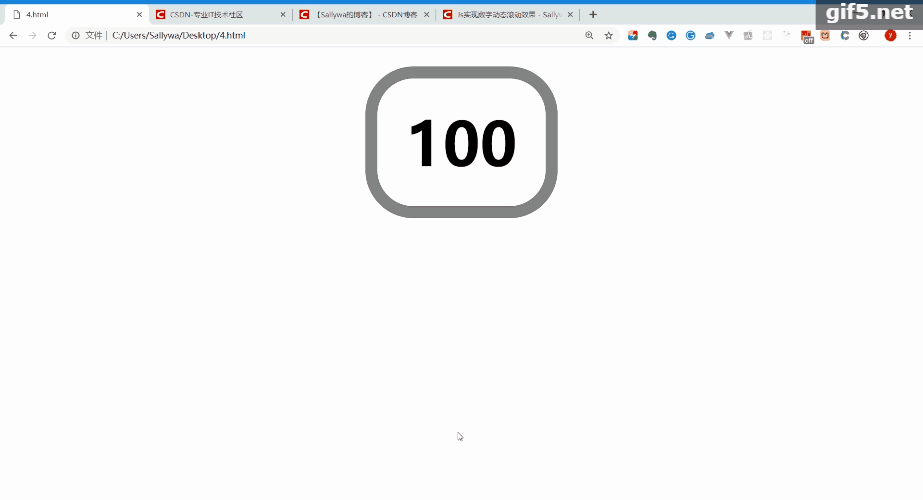完整代码如下

DOM

<!DOCTYPE html>
<html>
<title></title>
<style type="text/css">
#root{
display: flex;
justify-content: center;
width: 50px;
font-size: 25px;
font-weight: 600;
border: 5px solid grey;
}
</style>
<body>
<div id="root">0</div>
</body>

JS

start函数用于启动动画，roll函数是具体进行数字滚动的函数，它返回一个闭包用于存储变化的数组n（也就是实现的数字）。参数total是你所设置的最后停止的数字，step是你所希望的跳动的间隔。

start函数里面的runtime表示动画总共经历的时间，默认设置为1000ms，最小须大于等于300ms，避免动画过快看不清。

<script type="text/javascript">
function roll(total, idname, step) {
let n = 0;
return function () {
n = (n + step) >= total ? total : (n + step);
if (n <= total) {
document.getElementById(idname).innerHTML = n;
}
}
}
function start(index, idname, step, runtime = 1000){
let rolling = roll(index, idname, step)
runtime = (runtime >= 300) ? runtime : 1000;
for (let i = 0; i < (index/step); i++) {
let timer = setTimeout(rolling, (runtime/index)*i*step)
}
clearTimeout(timer);
}
start(100,'root', 4,)
</script>


展开全文• 滚动数字 创建节点 滚动原理 编写脚本 本节我们来实现数字滚动的效果。 运行效果如下： Cocos Creator版本：2.2.0 公众号后台回复"滚动数字"，获取完整项目源码： 创建节点 在层级管理器中我们创建...
滚动数字

创建节点

滚动原理

编写脚本

本节我们来实现数字滚动的效果。

运行效果如下：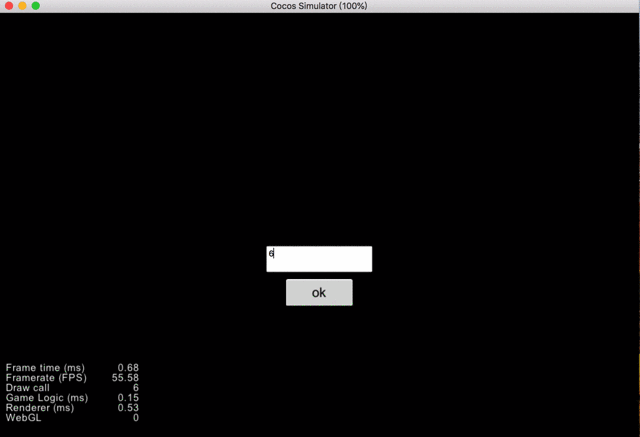Cocos Creator版本：2.2.0

公众号后台回复"滚动数字"，获取完整项目源码：创建节点

在层级管理器中我们创建以下节点：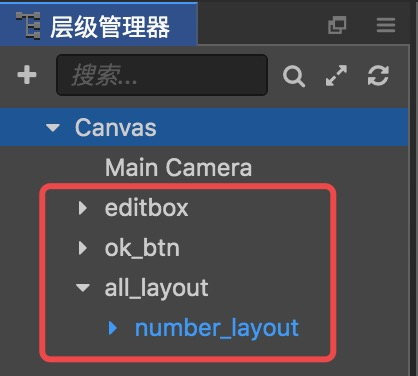esitbox即EditBox控件，用于输入数字。
ok_btn即一个按钮控件，当玩家输完数字，点击按钮后，数字滚动。
all_layout是一个横向布局节点，具体用处之后会介绍。
number_layout是本节教程的核心，它是一个垂直布局节点，其中放入值为0-9以及"."符号的这些Label控件。
number_layout节点：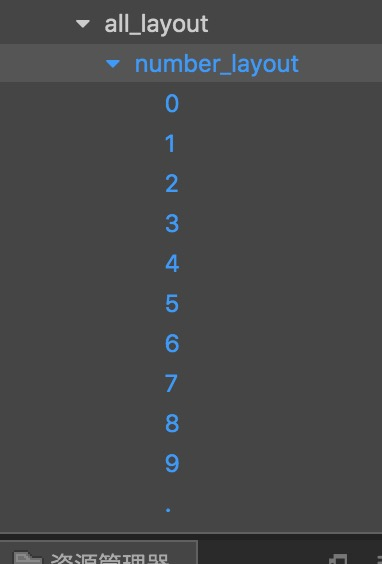注：水平和垂直布局的缩放模式(Resize Mode)都为CONTAINER。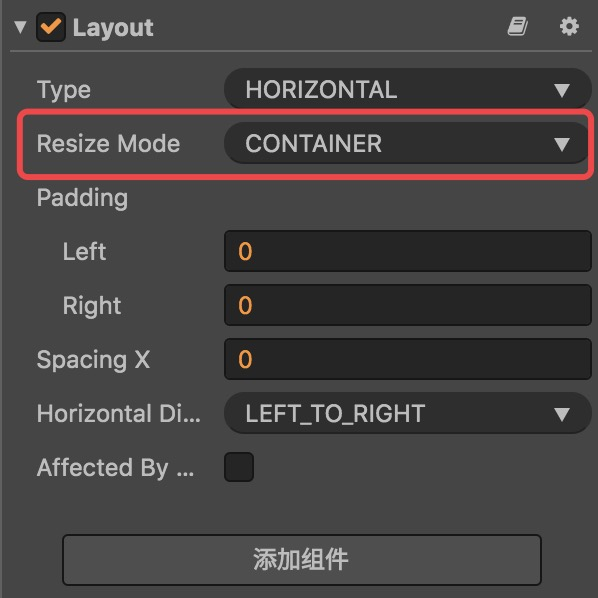此时场景编辑器显示如下：

注：节点的坐标大家可以自己摆放，或者打开笔者的项目查看，这里就不再截图。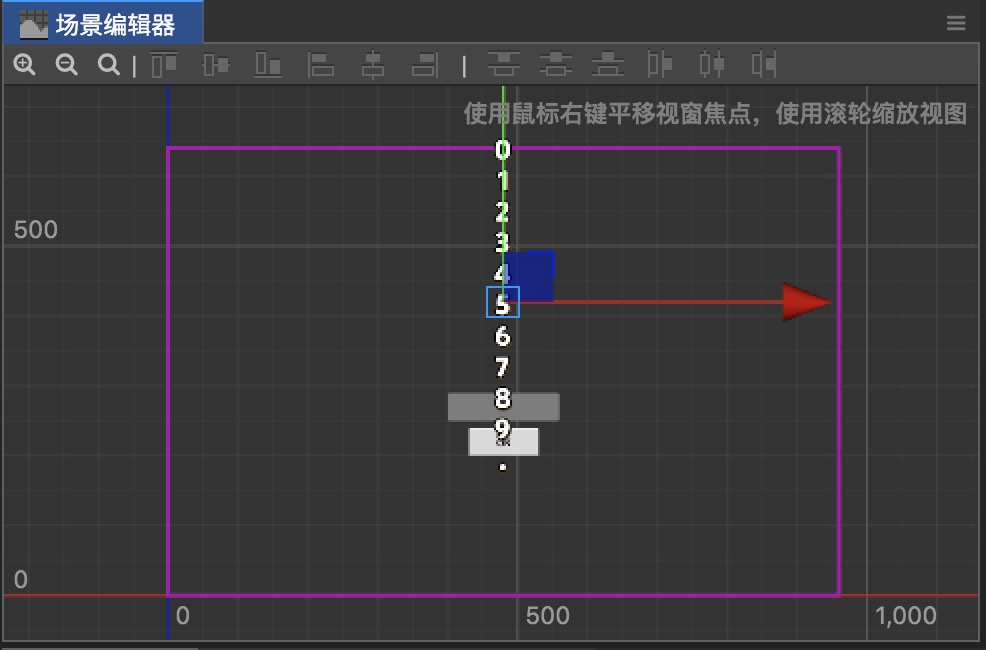滚动原理

其实数字滚动的原理非常简单，就是改变number_layout节点的y坐标。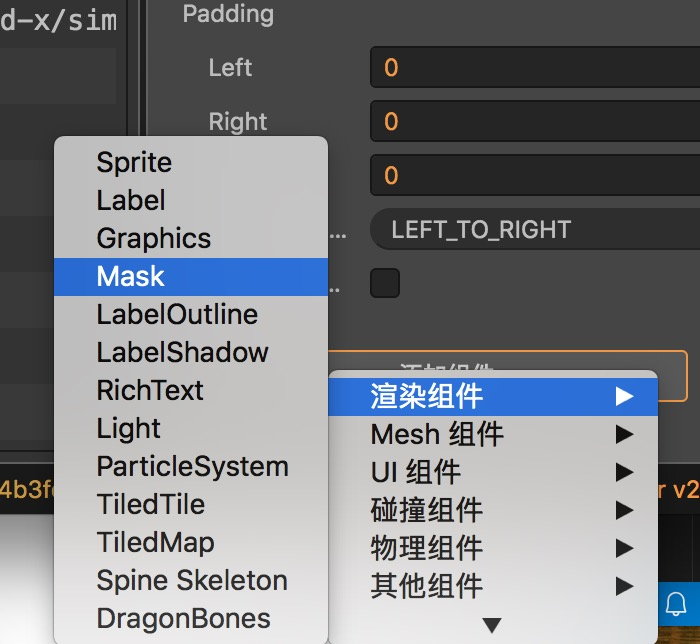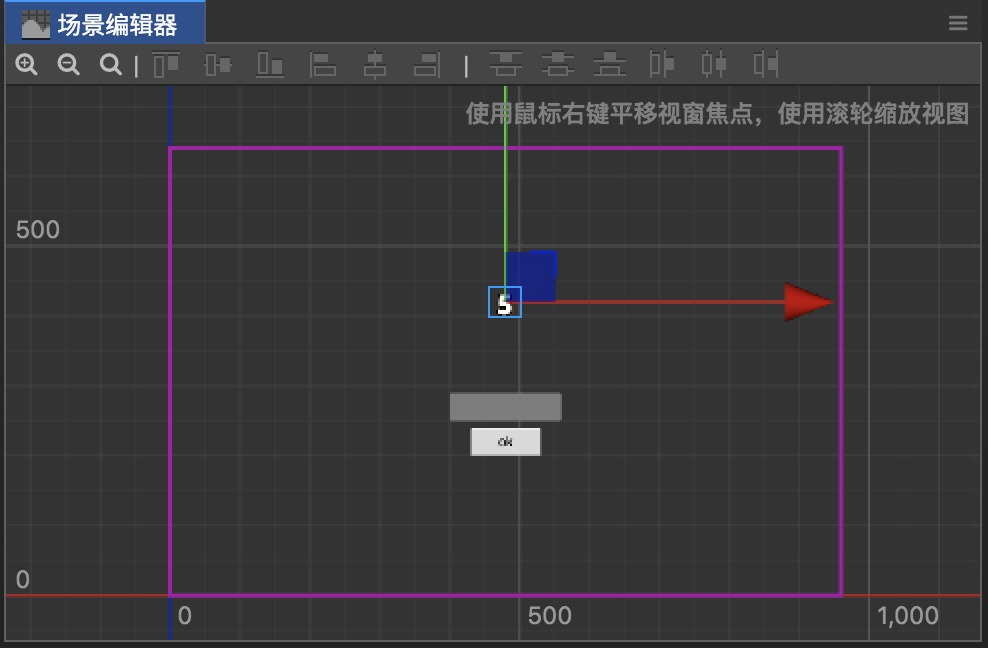那现在笔者把number_layout节点的y坐标变大，也就是让整个节点往上走，显示出来的就是比5大的数字了：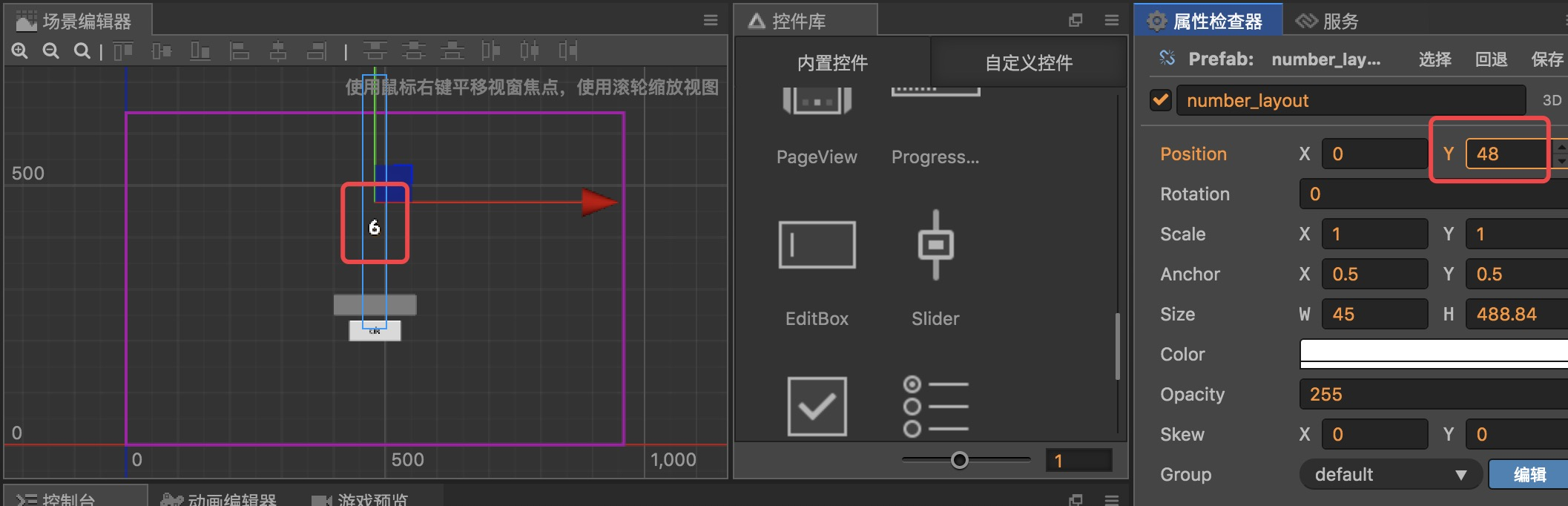同理，如果笔者把y坐标变小，显示的就会是比5小的数字。

number_layout代表的就是数字上某一位，如果要表示多位数，那么我们只用多创建几个number_layout就行了(自然而然想到应该把number_layout变为预制)。

编写脚本

我们创建一个名称为Roll.js的脚本，在properties中添加如下属性：

// Roll.js
properties: {
numLayoutPrefab: cc.Prefab,
allLayoutNode: cc.Node,
editNode: cc.Node,
rollTime: 2,
},

numLayoutPrefab就是number_layout变成的预制。
allLayoutNode就是all_layout节点。
editNode就是editbox节点。
rollTime为滚动动作所需时间。

// Roll.js
// 节点池
this.prefabPool = new cc.NodePool();
// 预制数组，方便管理
this.prefabArray = [];
// 获取每个数字长度，用于获取y坐标
let numLayout = cc.instantiate(this.numLayoutPrefab);
this.eachHeight = numLayout.height / 11;
this.prefabPool.put(numLayout);
},

节点池用来提高性能。
这里的prefabArray挺重要的，我们之后会讲到它的用法。
为了准确地显示出数字，我们需要求出number_layout中每个数字的高度(即每个Label控件的高度)，这样就可以求出number_layout目标坐标点的y值了。

现在我们实现getNumStr方法来获取输入框文本：

// Roll.js
getNumStr () {
// 获取编辑框中输入的数字(字符串)
let numStr = this.editNode.getComponent(cc.EditBox).string;
return numStr;
},

接着在okBtn方法中判断玩家输入的字符串是否可以转为数字(记得给ok_btn按钮绑上该方法)：

// Roll.js
okBtn () {
// 首先获取数字(字符串)
let numStr = this.getNumStr();

// 再判断是否可以转换为数字
let num = Number(numStr);
if (!isNaN(num)) {
console.log('是数字！');
}
else {
console.log('非数字！');
}
},

当确认玩家输入的是数字后，我们就可以生成相应位数的数字，并执行滚动效果。

数字的位数可以直接通过numStr的长度来判断，有多少位我们就生成多少个预制：

// Roll.js
okBtn () {
// 首先获取数字(字符串)
let numStr = this.getNumStr();

// 再判断是否可以转换为数字
let num = Number(numStr);
if (!isNaN(num)) {
// 根据numStr长度生成相应数量的预制
for(let i=0; i<numStr.length; i++) {
// 将生成的预制放入数组中
this.prefabArray.push(this.getNewPrefab());
}
}
else {
console.log('非数字！');
}
},

我们注意到每个通过getNewPrefab()生成的预制都被放到了prefabArray数组中。其实该数组变量的作用就是为了更好地管理各个预制(比如后期删除)。在这里我们还可以通过该数组控制数字上的各个位数——数组的第一个元素就是数字的最高位。

生成预制的getNewPrefab方法实现如下：

// Roll.js
getNewPrefab () {
// 生成预制
let numLayout = null;
// 根据节点池大小判断是从预制池中获取还是新生成一个
if (this.prefabPool.size() > 0)
numLayout = this.prefabPool.get();
else
numLayout = cc.instantiate(this.numLayoutPrefab);
// 添加到总布局中
return numLayout;
},

预制生成后就是执行滚动效果了：

// Roll.js
okBtn () {
...

if (!isNaN(num)) {
// 根据numStr长度生成相应数量的预制
for(let i=0; i<numStr.length; i++) {
// 将生成的预制放入数组中
this.prefabArray.push(this.getNewPrefab());
}

// 执行滚动效果
for(let i=0; i<numStr.length; i++) {
this.roll(numStr[i], this.prefabArray[i])
}
}
else {
console.log('非数字！');
}
},

roll方法实现如下：

// Roll.js
roll (num, prefab) {
// 关键是获取y坐标
let y = null;

if (num != '.')
y = (Number(num)-5)*this.eachHeight;
else
y = (10-5)*this.eachHeight;

let moveTo = cc.moveTo(this.rollTime, cc.v2(0, y)).easing(cc.easeCubicActionOut());
prefab.runAction(moveTo);
}

该方法有两个参数，一个是num，一个是 prefab。前者就是要显示的数字或"."符号，后者即相应位数上的number_layout预制。

我们首先判断num值，如果不是"."符号的话，那我们将调用Number()将该字符转为数字，减去5(5的y坐标为0)，再乘以每个数字的高度；是"."符号的话就用直接用10减去5再乘以数字高度("."符号排在最后个)。这样我们就得出了目标点的y坐标，接着将number_layout移到相应位置就可以了。

最后我们还要对okBtn()进行完善。每次点击ok按钮后，我们应当对之前生成的各个预制进行回收：

// Roll.js
okBtn () {
// 删除节点
this.allLayoutNode.removeAllChildren();

// 恢复坐标，回收预制，清空数组
for(let i=0; i<this.prefabArray.length; i++) {
this.prefabArray[i].setPosition(0, 0);
this.prefabPool.put(this.prefabArray[i]);
}
this.prefabArray = [];

...
},

注意要删除之前的节点，并将prefabArray数组清空，各个预制回收前也要恢复坐标(可以自行考虑下为什么)。

现在将Roll.js脚本挂到Canvas节点上，拖入相应节点，然后就可以运行项目啦：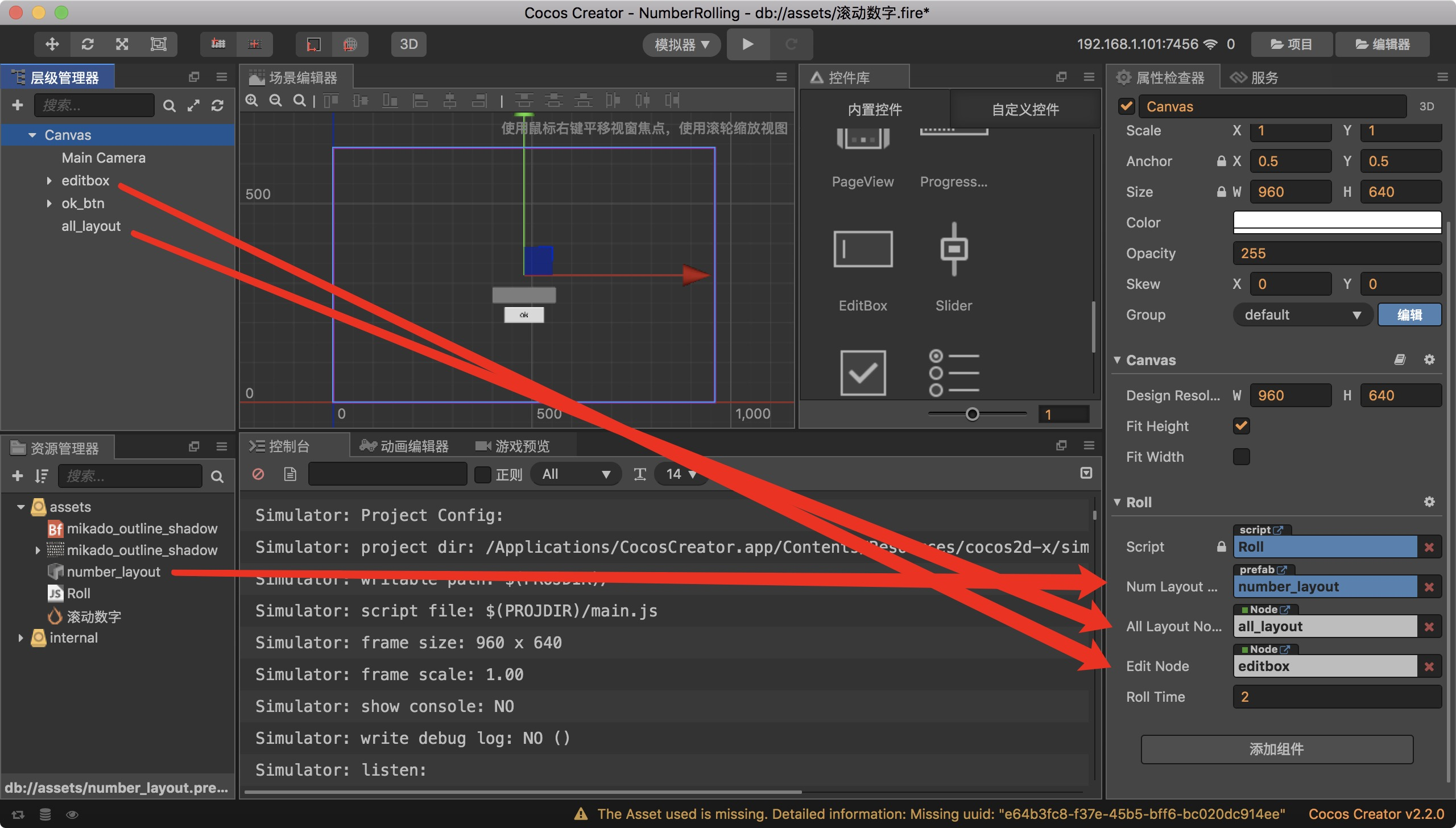那本期教程就到这，希望大家有所收获！

欢迎关注我的微信公众号，发现更多有趣内容：展开全文• H5页面特效 包扣时钟，动态路径，统计图，数字滚动等特效
• 动态数码显示两位数字，左右滚动显示
什么是动态数码管？
所谓的动态数码管不过是利用人的视觉残留效果，在多位显示数字。
20ms内在另一位数码管上显示数字，视觉上就像是同时点亮了两个数码管。而间隔时间过短则会造成亮度变暗。一般情况下为几ms间隔时间。
显示数字 16

程序现象：选择两位来显示数字
程序说明：1、573的D1~D8连接单片机的P0~P7。2、138的ABC引脚连接单片机P2.0,P2.1,P2.2;

#include "reg52.h"

sbit HC_A=P2^0;
sbit HC_B=P2^1;
sbit HC_C=P2^2;

int smgduan={0x3f,0x06,0x5b,0x4f,0x66,0x6d,0x7d,0x07,
0x7f,0x6f,0x77,0x7c,0x39,0x5e,0x79,0x71};//显示0~F的值

void delay(unsigned int i)    // 10*i us
{
while(i--);
}

void Select(unsigned int Pos)  // 位选，即选通某一个数码管
{
switch(Pos)
{
case(0):
HC_A=0;HC_B=0;HC_C=0; break;
case(1):
HC_A=1;HC_B=0;HC_C=0; break;
case(2):
HC_A=0;HC_B=1;HC_C=0; break;
case(3):
HC_A=1;HC_B=1;HC_C=0; break;
case(4):
HC_A=0;HC_B=0;HC_C=1; break;
case(5):
HC_A=1;HC_B=0;HC_C=1; break;
case(6):
HC_A=0;HC_B=1;HC_C=1; break;
case(7):
HC_A=1;HC_B=1;HC_C=1; break;
}

}

void show_two(int num,int pos_x,int pos_y)  // 选择显示两位数字的数码管位置
{
Select(pos_x);
P0=smgduan[num/10];
delay(100);
P0=0x00;

Select(pos_y);
P0=smgduan[num%10];
delay(100);
P0=0x00;
}

void main()
{
while(1)
{
show_two(16,6,7);
}

}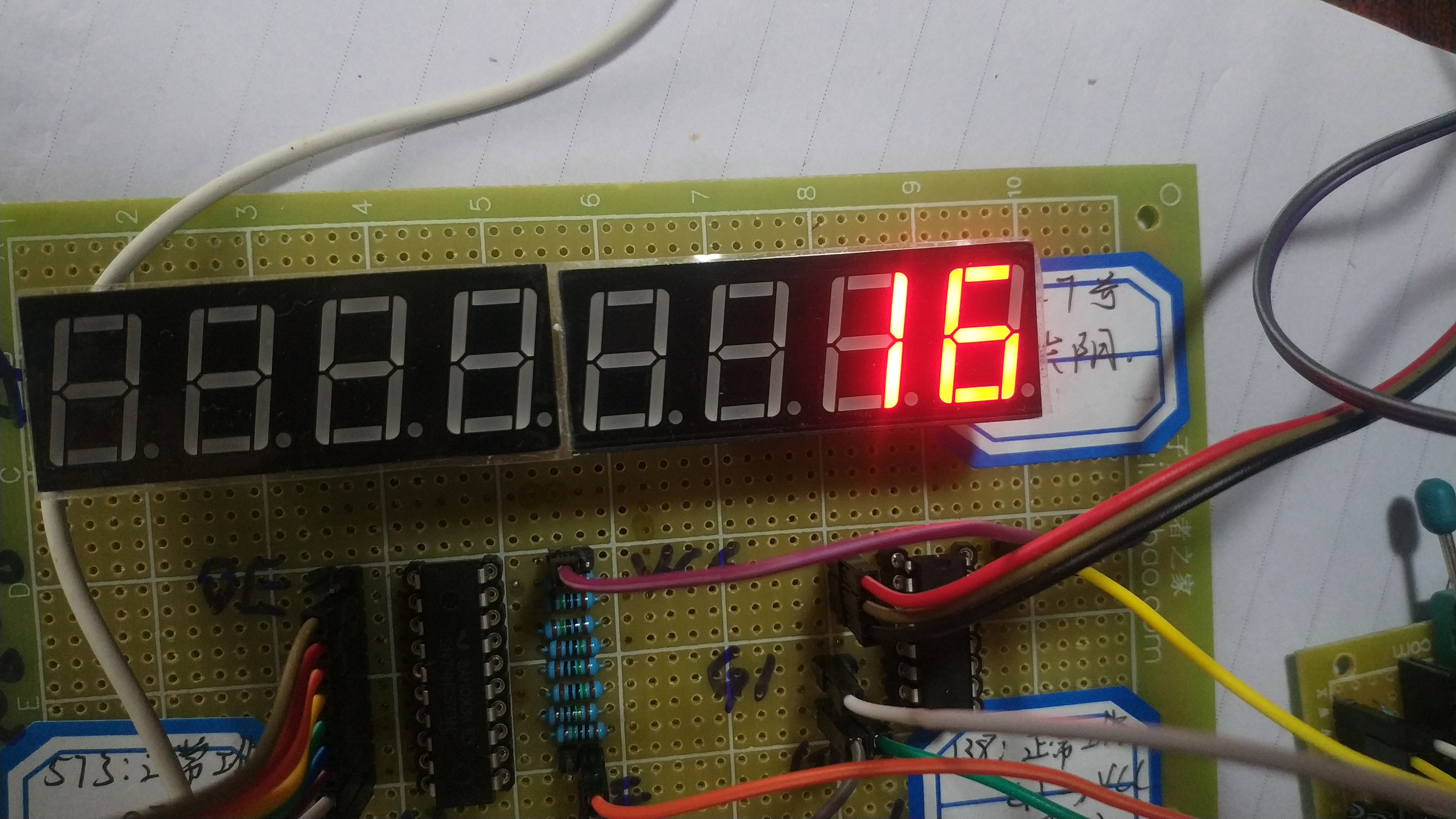左右滚动显示数字

程序现象：左移显示0到F，再右移显示F到0


#include "reg52.h"

sbit HC_A=P2^0;
sbit HC_B=P2^1;
sbit HC_C=P2^2;

int smgduan={0x00,0x00,0x00,0x00,0x00,0x00,0x00,0x00,
0x00,0x3f,0x06,0x5b,0x4f,0x66,0x6d,0x7d,0x07,
0x7f,0x6f,0x77,0x7c,0x39,0x5e,0x79,0x71,0x00,
0x00,0x00,0x00,0x00,0x00,0x00,0x00};//显示0~F的值,前面8个0，后面8个0

void delay(unsigned int i)    // 10*i us
{
while(i--);
}

void main()
{
int j=0,i=0;

for(j=0;j<33;j++)          //   左移，显示0~~F
{
for(i=0;i<50;i++)
{
HC_A=0;HC_B=0;HC_C=0;
P0=smgduan[j];  delay(100);P0=0x00;
HC_A=1;HC_B=0;HC_C=0;
P0=smgduan[j+1];delay(100);P0=0x00;
HC_A=0;HC_B=1;HC_C=0;
P0=smgduan[j+2];delay(100);P0=0x00;
HC_A=1;HC_B=1;HC_C=0;
P0=smgduan[j+3];delay(100);P0=0x00;
HC_A=0;HC_B=0;HC_C=1;
P0=smgduan[j+4];delay(100);P0=0x00;
HC_A=1;HC_B=0;HC_C=1;
P0=smgduan[j+5];delay(100);P0=0x00;
HC_A=0;HC_B=1;HC_C=1;
P0=smgduan[j+6];delay(100);P0=0x00;
HC_A=1;HC_B=1;HC_C=1;
P0=smgduan[j+7];delay(100);P0=0x00;
}
}
for(j=33;j>7;j--)   //   右移，显示F~~0
{
for(i=0;i<50;i++)
{
HC_A=1;HC_B=1;HC_C=1;
P0=smgduan[j-1];delay(100);P0=0x00;
HC_A=0;HC_B=1;HC_C=1;
P0=smgduan[j-1];delay(100);P0=0x00;
HC_A=1;HC_B=0;HC_C=1;
P0=smgduan[j-2];delay(100);P0=0x00;
HC_A=0;HC_B=0;HC_C=1;
P0=smgduan[j-3];delay(100);P0=0x00;
HC_A=1;HC_B=1;HC_C=0;
P0=smgduan[j-4];delay(100);P0=0x00;
HC_A=0;HC_B=1;HC_C=0;
P0=smgduan[j-5];delay(100);P0=0x00;
HC_A=1;HC_B=0;HC_C=0;
P0=smgduan[j-6];delay(100);P0=0x00;
HC_A=0;HC_B=0;HC_C=0;
P0=smgduan[j-7];delay(100);P0=0x00;
}
}
while(1);

}




展开全文• 1、前言：最近的项目需求中，对有些数字的变化，客户提了需求，加一些加载效果用户体验会更好。所以用了vue-animate-number 这一款插件 2、案例:https://wangdahoo.github.io/vue-animate-number vue-animate-...
• 最近在做个小软件，用到定时提醒功能，定时选择时间的时候我想到了锤子手机的闹钟设置界面，当然这种数字滚动选择器也是很常见啦，类似下面的效果 参考了　...
• jQuery滚动到页面底部后数字从0开始滚动到指定数字文字特效代码,包括小数点实现滚动，都可以实现你想要的效果！
• 业务中需要用到滚动数字，找了一些库jq比较多，还有数字是从0到9的滚动，比较出名的是countUp.js，而我自己想要的是数字上下滚动，就自己动手写了一下，效果如下： npm下载地址： vue版：count-up-number ...vue react npm
• 主要为大家详细介绍了vue实现数字滚动效果，文中示例代码介绍的非常详细，具有一定的参考价值，感兴趣的小伙伴们可以参考一下
• 之前看的案例都是列表滚动，所以自己写了一个文本动态显示 <template> <div class="marquee_box" ref="boxsize"> <p class="marquee_text" ref="boxtext" style="padding:6px;margin-top:...vue 显示 文本
• 一开始我是直接从网上找了一个插件，但是这个插件并不是匀速滚动的，这就导致当页面刷新后显示的数字不是刷新之前的，这样肯定不行啊，那么我们就思考将24小时分成若干等份来计算，下面直接贴代码： // 滚动效果...
• 因为需要，自己用jquery动手写了数字滚动效果 实现原理 1、先确定数字最大的位数，比如说最大数为6位数，就画出一排6个高度为30px的方格; 2、然后在每个方格区域增加一竖排的 0123456789，定时更新数字，转成字符...js
• 在很多地方我们能看到一些数字变换比较快的数字滚动器，一般数字滚动器有两种，一种是一个值一直在原位发生改变，另一种则是每个数字都有自己的位置，当发生变化时向上或者向下有0-9的滚动效果，今天我们来讨论第一...input
• https://github.com/inorganik/countUp.js target= 目标元素的 ID； startVal= 开始值； endVal= 结束值； decimals= 小数位数，默认值是0； duration= 动画延迟秒数，默认值是2；...vardemo =new...
• &lt;script src="http://www.zzwtqx.com/index/js/jquery-1.4.2.min.js"&gt;&lt;/script&gt; &lt;div style="margin-top=2000px;background="#ccc"...n
• 金币数字滚动增加 //星星动态更新 updateStarLabel: function (num) { let timeCount = 10; let star = userData.star; let starLabel = this.homeUI_js.starLabel; let callback = function () { timeCount-...
• ## 实现HTML数字滚动

千次阅读 2018-12-14 15:39:18
轻量级jquery数字动画插件|DEMO_jQuery之家-自由分享jQuery、html5、css3的插件库          .container { margin:150px auto; max-width:480px;}  h2,h3 { text-align:center;}  .demo { text-align...html jquery js
• 为了实现数字的无限滚动效果，每个数字框的内部，其实包含了两组0~9的view，每个View的高度都一样 数字框内使用绝对定位，通过调整top位置，显示出指定的数字 使用transtion动画，top发生变化时就会滚动，然后通过...微信小程序...